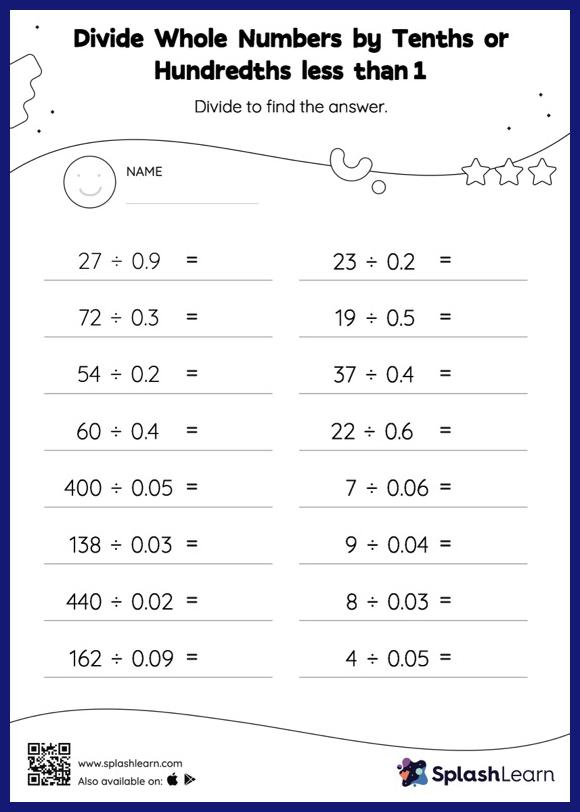# Divide Whole Numbers by Tenths or Hundredths less than 1: Horizontal Division Worksheet

Home > Divide Whole Numbers by Tenths or Hundredths less than 1: Horizontal DivisionThis worksheet strengthens students' accuracy and strategic flexibility while they divide whole numbers by tenths or hundredths less than 1. Students divide a whole number by a decimal without taking the decimal point into account, and then, depending on the number of decimal digits in the divisor, they move each digit of the quotient to the left. Students practice this concept in great depth with divide whole numbers by tenths or hundredths less than 1 worksheet. In this worksheet, students practice solving problems written in the horizontal format. How numbers are laid out in a problem affects the method a student employs to solve it. Therefore to develop actual fluency and mastery of multiple strategies, students must practice different formats.